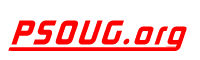Quick Search: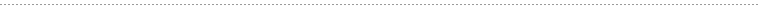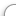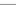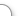CODE Oracle PL/SQL Code Library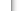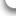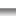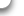JOBS Find Or Post Oracle JobsFORUM Oracle Discussion & ChatPHP Code: Calculate File Size In Directory Jump to: Select a Location PSOUG Home Page Oracle Code Library mySQL Code Library PHP Code Library JavaScript Code Library Oracle Terms & Definitions Oracle Error Codes PSOUG Community Blogs Oracle Jobs Board PSOUG Forum Oracle User Group Directory Free Oracle Magazines Online Learning Center PSOUG Presentations Advanced Code Search News and Events Sponsors Page Submit Code Contact UsSnippet Name: Calculate File Size In Directory

Description: This is a PHP Class that calculates the size, number of files & folders of a specific directory.

Comment: (none)

Language: PHP
Highlight Mode: PHP

```<?PHP

// calculate.directory.class.php
// Credits: BitRepository.com
// URL: http://www.bitrepository.com/web-programming/php/
// calculate-the-size-number-of-files-folders-of-a-directory.html

CLASS Directory_Calculator {

VAR \$size_in;
VAR \$decimals;

FUNCTION calculate_whole_directory(\$directory)
{
IF (\$handle = OPENDIR(\$directory))
{
\$size = 0;
\$folders = 0;
\$files = 0;

WHILE (FALSE !== (\$file = READDIR(\$handle)))
{
IF (\$file != "." && \$file != "..")
{
IF(IS_DIR(\$directory.\$file))
{
\$array = \$this->calculate_whole_directory(\$directory.\$file.'/');
\$size += \$array['size'];
\$files += \$array['files'];
\$folders += \$array['folders'];
}
ELSE
{
\$size += FILESIZE(\$directory.\$file);
\$files++;
}
}
}
CLOSEDIR(\$handle);
}

\$folders++;

RETURN ARRAY('size' => \$size, 'files' => \$files, 'folders' => \$folders);
}

FUNCTION size_calculator(\$size_in_bytes)
{
IF(\$this->size_in == 'B')
{
\$size = \$size_in_bytes;
}
ELSEIF(\$this->size_in == 'KB')
{
\$size = ((\$size_in_bytes / 1024));
}
ELSEIF(\$this->size_in == 'MB')
{
\$size = ((\$size_in_bytes / 1024) / 1024);
}
ELSEIF(\$this->size_in == 'GB')
{
\$size = ((\$size_in_bytes / 1024) / 1024) / 1024;
}

\$size = ROUND(\$size, \$this->decimals);

RETURN \$size;
}

FUNCTION size(\$directory)
{
\$array = \$this->calculate_whole_directory(\$directory);
\$bytes = \$array['size'];
\$size = \$this->size_calculator(\$bytes);
\$files = \$array['files'];
\$folders = \$array['folders'] - 1; // exclude the main folder

RETURN ARRAY('size'    => \$size,
'files'   => \$files,
'folders' => \$folders);
}
}
?>

Here&#39;s an usage example of this class:

example.php

<?PHP
SET_TIME_LIMIT(10000);

INCLUDE 'calculate.directory.class.php';

/* Path to Directory - IMPORTANT: with '/' at the end */

\$directory = '/home/mywebsite.com/public_html/';

/* Calculate size in: B (Bytes), KB (Kilobytes), MB (Megabytes), GB (Gigabytes) */

\$size_in = 'MB';

/* Number of decimals to show */

\$decimals = 2;

\$directory_size = NEW Directory_Calculator;

/* Initialize Class */

\$directory_size->size_in = \$size_in;
\$directory_size->decimals = \$decimals;

// return an array with: size, total files & folders
\$array = \$directory_size->size(\$directory);

ECHO "The directory <em>".\$directory."</em> has a size of
".\$array['size']." ".\$size_in.", ".\$array['files']." files &
".\$array['folders']." folders.";
?>```FreeOracle MagazineSubscriptionsand Oracle White Papers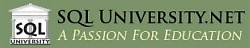SQL University.net courses meet the most demanding needs of the business world for advanced education in a cost-effective manner. SQL University.net courses are available immediately for IT professionals and can be taken without disruption of your workplace schedule or processes.

Compared to traditional travel-based training, SQL University.net saves time and valuable corporate resources, allowing companies to do more with less. That's our mission, and that's what we deliver.